Next: Non-uniform circular motion Up: Circular motion Previous: Centripetal acceleration

## The conical pendulum

Suppose that an object, mass, is attached to the end of a light inextensible string whose other end is attached to a rigid beam. Suppose, further, that the object is given an initial horizontal velocity such that it executes a horizontal circular orbit of radiuswith angular velocity. See Fig. 60. Let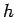be the vertical distance between the beam and the plane of the circular orbit, and letbe the angle subtended by the string with the downward vertical.The object is subject to two forces: the gravitational force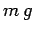which acts vertically downwards, and the tension force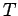which acts upwards along the string. The tension force can be resolved into a component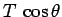which acts vertically upwards, and a componentwhich acts towards the centre of the circle. Force balance in the vertical direction yields(262)

In other words, the vertical component of the tension force balances the weight of the object.

Since the object is executing a circular orbit, radius, with angular velocity, it experiences a centripetal acceleration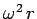. Hence, it is subject to a centripetal force. This force is provided by the component of the string tension which acts towards the centre of the circle. In other words,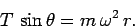(263)

Taking the ratio of Eqs. (262) and (263), we obtain(264)

However, by simple trigonometry,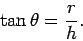(265)

Hence, we find(266)

Note that if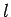is the length of the string then. It follows that(267)

For instance, if the length of the string is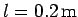and the conical angle is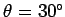then the angular velocity of rotation is given by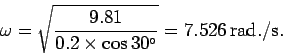(268)

This translates to a rotation frequency in cycles per second of(269)Next: Non-uniform circular motion Up: Circular motion Previous: Centripetal acceleration
Richard Fitzpatrick 2006-02-02